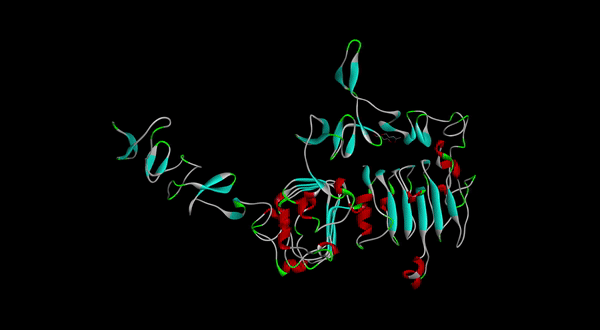# Team:GreatBay SCIE/ModelModeling Overview

# Introduction

Mathematical modeling is a useful tool in synthetic biology, as it allows us to describe the relationship between different parameters and variables during biochemical processes using a set of mathematical equations. In our project, we used mathematical modeling to obtain quantitative solutions to several problems we encounter in our project.

# Mathematical Modeling

In our first model, we have predicted the optimum concentration of the aptamer used for the nanoparticle-aptamer conjugation, to improve the aptamers' binding affinity by mainly applying the principles and theories of calculus. By doing so, the efficiency of the drug we use can be substantially increased.

In our second model, in order to predict the effectiveness of our drug, we have predicted the drug loading amount in a liposome by using Fick's Laws, which can assist the manufacturing of the drug and help predict the usage amount of the drug better.

Besides, we also construct an additional modeling of binding constant to see the binding affinity of our aptamer to the HER2